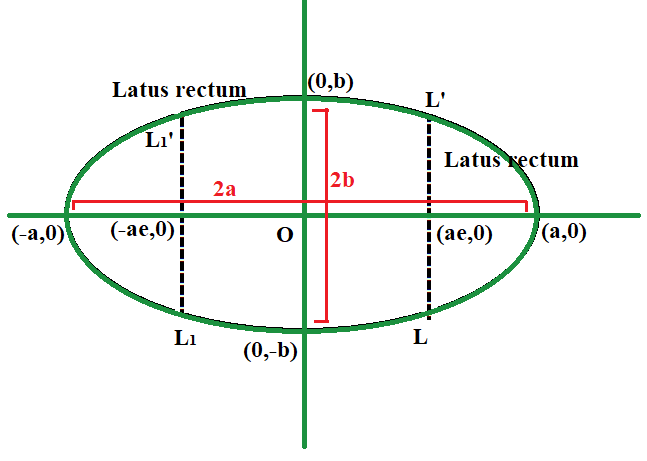GFG App
Open AppBrowser
Continue

# Program to find the Eccentricity of an Ellipse

Given two positive integers A and B, representing the length of the semi-major and semi-minor axis of an ellipse of the equation, the task is to find the eccentricity of the given ellipse.

Examples:

Input: A = 12, B = 9
Output: 0.66

Input: A = 6, B = 3
Output: 0.87

Approach: The given problem can be solved based on the following formula to calculate the eccentricity of an ellipse which is given by:where,
A = Length of semi major axis
B = Length of semi minor axisTherefore, print the value ofas the eccentricity of the ellipse.

Below is the implementation of the above approach:

## C++

 // C++ program for the above approach   #include  using namespace std;   // Function to find the // eccentricity of ellipse void findEccentricity(double A,                       double B) {     // Store the squares of length of     // semi-major and semi-minor axis     double semiMajor = A * A;     double semiMinor = B * B;       // Calculate the eccentricity     double ans = sqrt(1 - semiMinor / semiMajor);       // Print the result     cout << fixed << setprecision(2)          << ans; }   // Driver Code int main() {     double A = 12, B = 9;     findEccentricity(A, B);       return 0; }

## Java

 // Java program for the above approach import java.util.*;   class GFG{   // Function to find the // eccentricity of ellipse static void findEccentricity(double A, double B) {     // Store the squares of length of     // semi-major and semi-minor axis     double semiMajor = A * A;     double semiMinor = B * B;           // Calculate the eccentricity     double ans = Math.sqrt(1 - semiMinor / semiMajor);       // Print the result     System.out.format("%.2f", ans); }   // Driver Code public static void main(String arr[]) {     double A = 12, B = 9;     findEccentricity(A, B); } }   // This code is contributed by kirti

## Python3

 # Python3 program for the above approach import math   # Function to find the # eccentricity of ellipse def findEccentricity(A, B):           # Store the squares of length of     # semi-major and semi-minor axis     semiMajor = A * A     semiMinor = B * B       # Calculate the eccentricity     ans = math.sqrt(1 - semiMinor / semiMajor)       # Print the result     print('%.2f' % ans)   # Driver Code if __name__ == "__main__":       A = 12     B = 9           findEccentricity(A, B)       # This code is contributed by ukasp

## C#

 // C# program for the above approach using System;   class GFG{   // Function to find the // eccentricity of ellipse static void findEccentricity(double A, double B) {           // Store the squares of length of     // semi-major and semi-minor axis     double semiMajor = A * A;     double semiMinor = B * B;            // Calculate the eccentricity     double ans = Math.Sqrt(1 - semiMinor / semiMajor);        // Print the result     Console.Write(Math.Round(ans, 2)); }   // Driver code static void Main() {     double A = 12, B = 9;           findEccentricity(A, B); } }   // This code is contributed by code_hunt

## Javascript

 

Output:

0.66

Time Complexity: O(1)
Auxiliary Space: O(1)

My Personal Notes arrow_drop_up